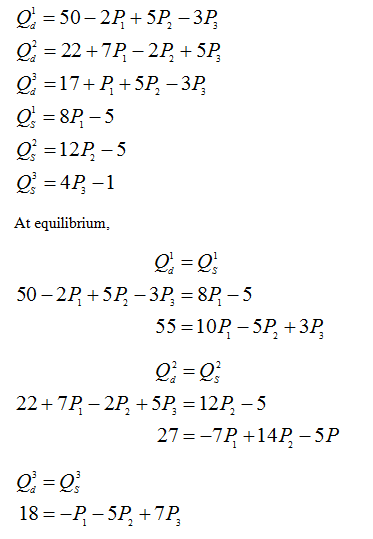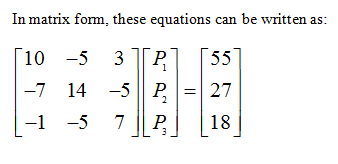# Use a matrix method to find the equilibrium prices and quantities where the supply and demand functionsfor Good 1, Good 2 and Good 3 are asQd1 = 50 − 2P1 + 5P2 − 3P3, Qs1 = 8P1 − 5Qd2 = 22 + 7P1 − 2P2 + 5P3, Qs2 = 12P2 − 5Qd3 = 17 + P1 + 5P2 − 3P3, Qs3 = 4P3 − 1

Question
51 views

Use a matrix method to find the equilibrium prices and quantities where the supply and demand functions
for Good 1, Good 2 and Good 3 are as
Qd1 = 50 − 2P1 + 5P2 − 3P3, Qs1 = 8P1 − 5
Qd2 = 22 + 7P1 − 2P2 + 5P3, Qs2 = 12P2 − 5
Qd3 = 17 + P1 + 5P2 − 3P3, Qs3 = 4P3 − 1

check_circle

Step 1

Following equation is given:...

### Want to see the full answer?

See Solution

#### Want to see this answer and more?

Solutions are written by subject experts who are available 24/7. Questions are typically answered within 1 hour.*

See Solution
*Response times may vary by subject and question.
Tagged in